Next: Testing of the SUSAN Edge Detector Up: The SUSAN Edge Detector Previous: The SUSAN Edge Detector in Detail

## Analysis of the SUSAN Edge Detector

A simple derivation is now given which shows the theoretical coincidence of the exact SUSAN edge position and the zero crossing of the second derivative of the image function.

If we assume a one dimensional input signal which is monotonically increasing then it can be simply shown that a minimum in the USAN area (in this case USAN length) will be equivalent to the edge definition which places the edge at the position of inflection of the curve, that is, where the second derivative of the image function is zero. See Figure 7 for an example image function and three one dimensional mask positions.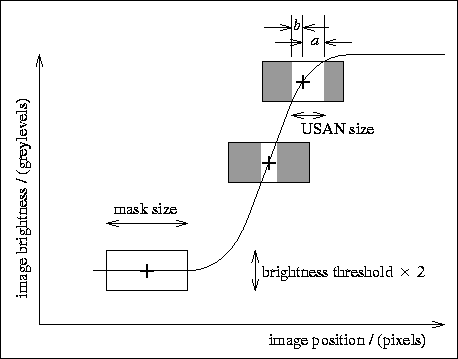Figure 7: A monotonically increasing one dimensional input signal and three mask positions showing USAN size varying with signal gradient. The USAN is shown as the white portion of the mask. The mask centred on the signal inflection has the smallest USAN.

If we have general image position x, image function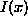and brightness threshold t, then a and b (the ``right'' and ``left'' widths of the USAN -- see Figure 7), for any specific image position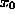, are, by definition, given by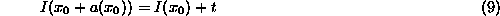and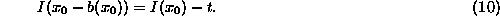The SUSAN principle is formulated in the following equation, where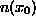is the USAN size at(the size being a length in this one dimensional case);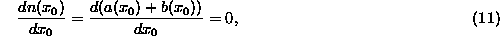assuming that a check is made for n being a minimum and not a maximum. This gives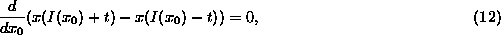giving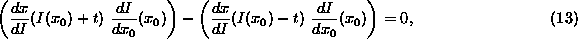that is,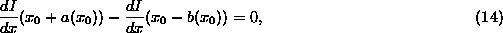unless the image function is constant, an uninteresting case. This is equivalent, in the continuous limit (that is, as t tends to zero), to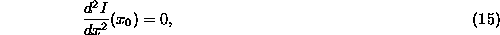and we have equivalence between the two definitions of exact edge position.

This mathematical equivalence in no way means that the SUSAN edge finding method is performing the same function as a derivative based edge detector. No derivatives are taken; indeed, no direction of maximum gradient is identified (in order for differentiation to be performed) in the first place. However, the preceding argument shows why the SUSAN edge detector gives edges to good sub-pixel accuracy even with edges that are not perfect step edges.

It can be seen from the preceding discussion that the initial response of the SUSAN edge detector to a step edge will be increased as the edge is smoothed. The response is also broadened.

As mentioned earlier, the form of the brightness comparison function, the heart of USAN determination (Equation 4), can be shown to achieve the optimal balance between stability and enhancement. This is equivalent to satisfying the criterion that there should be a minimum number of false negatives and false positives. This criterion is formulated in the following expression: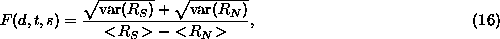where F is proportional to the number of false positives and false negatives that will be reported, s is image noise standard deviation,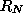is the SUSAN response strength with no edge present and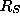is the SUSAN response strength when the mask is centred on an edge of strength d. Thus this expression simply divides the expected total ``noise'' by the expected ``signal'' in the most logical way.

The value of F will depend on the relative values of d, t and s. Thus fixing t at 1 and varying the other two variables will cater for all eventualities. If this expression is averaged over all likely values of d and s, the overall quality can be evaluated. The (wide) ranges of d and s chosen were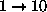and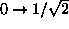respectively; the reason for choosing the the upper limit on s will shortly become apparent.

All of the calculations within the SUSAN feature detectors are based around the use of the equation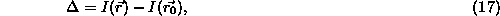where I is defined as before. If the image noise is Gaussian with standard deviation s then the noise on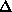will also be Gaussian with standard deviation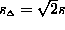. Thus F is evaluated over the interval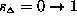.

To evaluate F given d, t and s, it is necessary to find the expectation values and variances ofand, using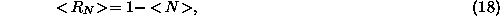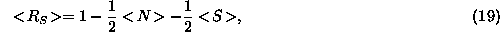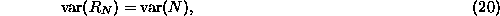and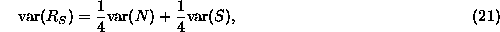where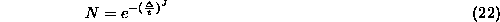and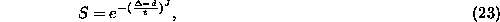with J being the even number determining the shape of the brightness comparison function; it is 2 for a Gaussian and infinity for a square function. (N and S are responses due to individual pixels with no edge present and across an edge respectively, using the brightness comparison function. The form ofarises from the fact that in the presence of an edge, half of the response will be due to noise only, and half of the response will be due to the edge as well as noise.) The expectation values and variances are computed using the integrals: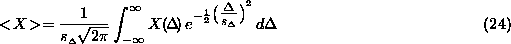andwhere the integral includes the Gaussian model of noise. The integrals are calculated over the intervals of d and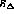specified and the mean result for F is thus found. The resulting plot of F against J is shown in Figure 8.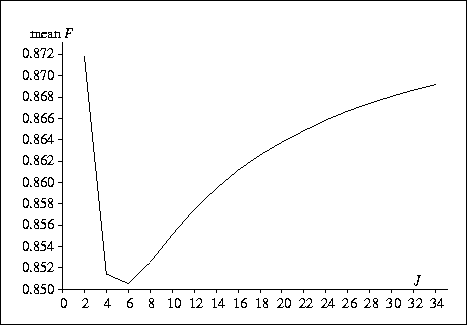Figure 8: A plot of an objective formulation of expected false negatives and positives against the J factor in the brightness comparison function.

It is clear that the optimal value for J is 6. This gives the form of the function used in the SUSAN filters.

The optimal value for g, the geometric threshold, is found by calculating the mean expectation value for N over the same realistic range of image noise as before. With no noise present,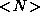is 1; its mean value in the presence of noise is calculated (using the integral shown in Equation 24) to be close to 0.75. Therefore the value of g which is likely to provide the correct amount of noise rejection is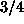of the maximum possible USAN size.

Finally, the spatial part of the filter is of interest. In  the ``difference of boxes'' edge detector is compared unfavourably with a ``derivative of Gaussian'' edge detector, due to the sharp cutoff in the spatial profile of the ``box'' filter. Because the Gaussian based filter has smooth edges, image noise is suppressed more effectively than using a box (or square) shaped filter. Therefore it may seem that a similar approach to the design of the mask used in SUSAN feature detection would be preferable. Indeed, in the brightness domain, it was found worthwhile to use a smooth brightness comparison function (as shown in Equation 4), rather than having thresholding done with a sharp cutoff. In the case of the spatial extent of the mask, the equivalent process would be achieved by using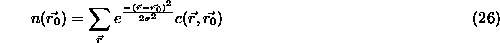in place of Equation 2, whereis a suitable scaling factor. This would give a circular mask with pixels contributing less to the calculations as their distance from the nucleus increased -- a Gaussian profile. An edge detector based on this kind of mask has been fully implemented and tested.

The difference in practice between the Gaussian spatial SUSAN detector and a sharp cutoff SUSAN detector turns out to be minimal; there is very little difference in the final reliability or localization of reported edges. The explanation for this possibly surprising result is that, unlike the differential edge detector, no image derivatives are used, so that the problem of noise is vastly reduced in the first place. Also, a linear filter is performing a different task to the initial SUSAN response. In the former, a filter has a Gaussian profile in a ``brightness versus distance'' plot. However, the latter uses either a sharp circular mask or a Gaussian profile in the spatial domain and smoothed thresholding in the brightness domain. Thus ``half'' of the algorithm already has a smooth cutoff action. From the results, it seems that it is this part (the brightness domain) which is more important. Thus it does not appear necessary to use a Gaussian spatial mask, although it is easy to do so if desired.Next: Testing of the SUSAN Edge Detector Up: The SUSAN Edge Detector Previous: The SUSAN Edge Detector in Detail

LaTeX2HTML conversion by Steve Smith (steve@fmrib.ox.ac.uk)